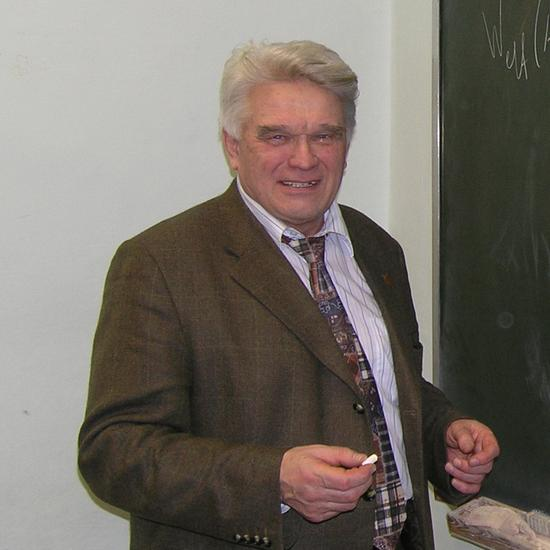Foreign Member

Elected: 2010

## Biography

Ludwig Faddeev was a mathematical physicist renowned for his contributions to quantum theory — how scientists understand the interactions of fundamental particles. In particular, he was known for the Faddeev equations, which describe all possible interactions of a system of three bodies using quantum mechanics. Ludwig’s work has also opened up new fields for exploration by mathematicians.

Ludwig’s research was rooted in his belief that mathematical elegance is an important guiding principle in physics, and that a ‘good’ problem in physics should yield new insight in pure mathematics. This approach enabled Ludwig to apply mathematical techniques from one area of physics to entirely new ones. For example, the emergence of the field of quantum groups was stimulated by the connections he made.

The importance of Ludwig’s work has been recognised with awards for both physics and mathematics. These include the 1975 Dannie Heineman Prize for Mathematical Physics of the American Physical Society, the 2006 Henri Poincaré Prize for outstanding contributions in mathematical physics, and the 2008 Shaw Prize in Mathematical Sciences.

Professor Ludwig Faddeev ForMemRS died on 26 February 2017.

## Awards

• #### Shaw Prize

For contributions to mathematical physics.